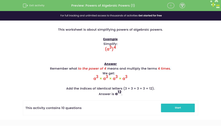# Multiply Algebraic Powers

In this worksheet, students must simplify powers of algebraic powers.Key stage:  KS 3

Curriculum topic:   Algebra

Curriculum subtopic:   Simplify Algebraic Expressions to Maintain Equivalence

Popular topics:   Algebra worksheets

Difficulty level:#### Worksheet Overview

This activity is about simplifying algebraic powers.

Example

Simplify:

(a3)4

Remember what to the power of 4 means and multiply the terms 4 times.

We get:

a3 × a3 × a3 × a3

Add the indices of identical letters (3 + 3 + 3 + 3 = 12).

A quicker method is to simply multiply together the two powers in the original term:

3 x 4 = 12, so the answer is a12

Let's have a go at some questions now.

### What is EdPlace?

We're your National Curriculum aligned online education content provider helping each child succeed in English, maths and science from year 1 to GCSE. With an EdPlace account you’ll be able to track and measure progress, helping each child achieve their best. We build confidence and attainment by personalising each child’s learning at a level that suits them.

Get started